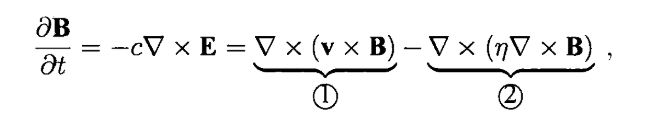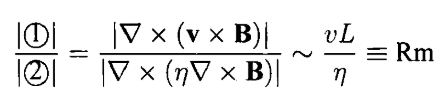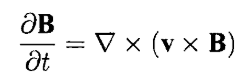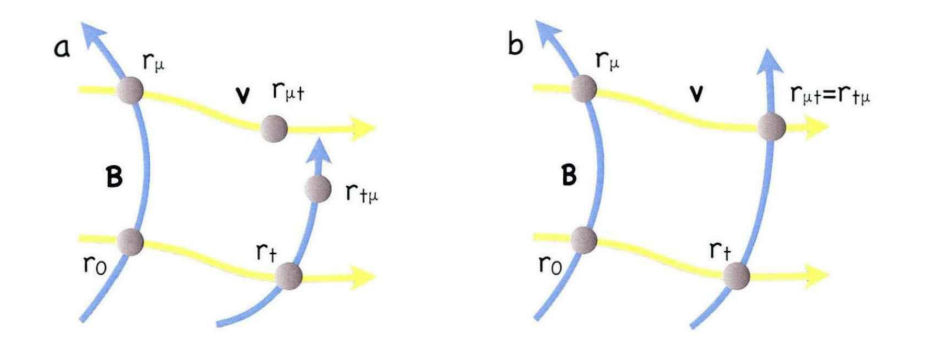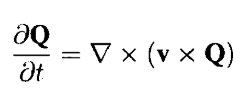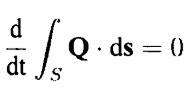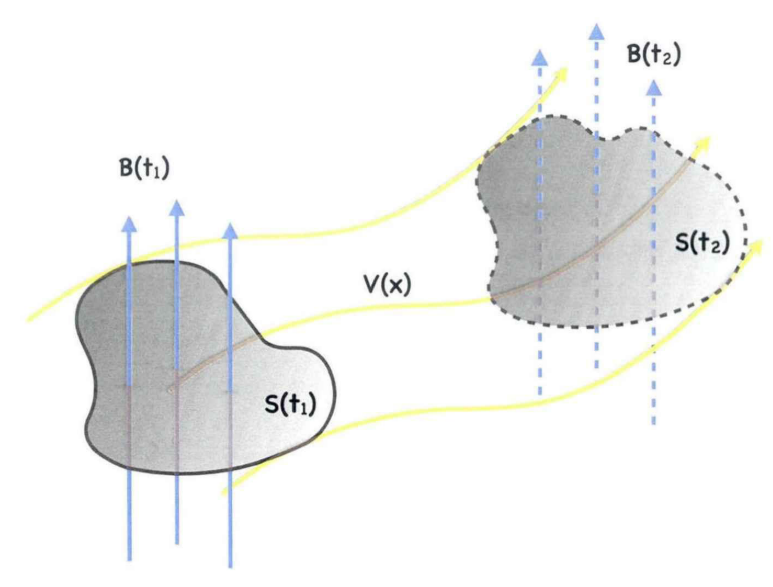# 磁场拓扑理论简介

2.1 磁场连接性

dr/ds=B(r(s))/|B(r(s))|

dr/dµ=B(r(µ))/|ρ(r(µ))B(r(s))|

Φtube=∫SB⋅ds

E=-v/c×B+c/(4πσ)∇×B